# Calorific Value Lecture 3, Fuel Tech-ll.pptx

20 Mar 2023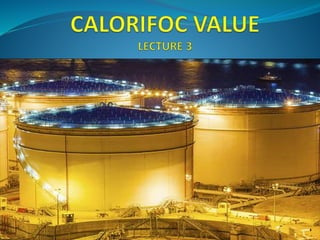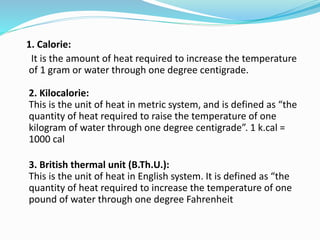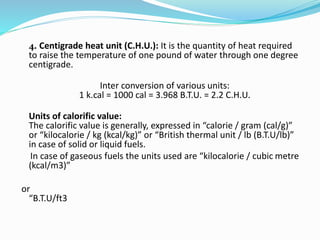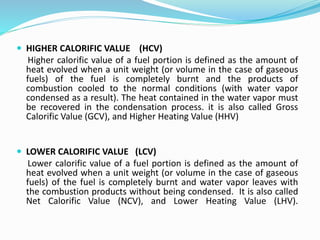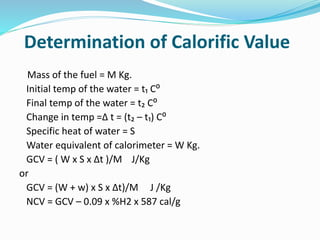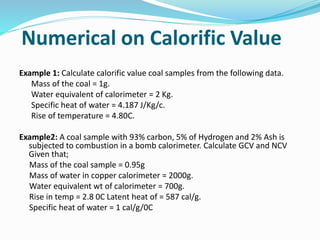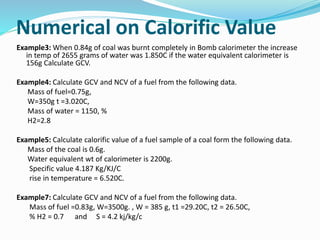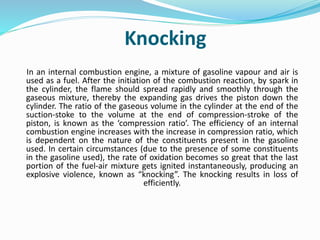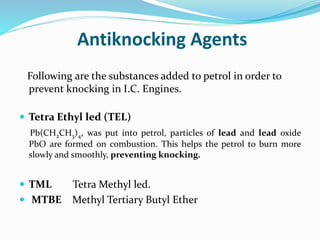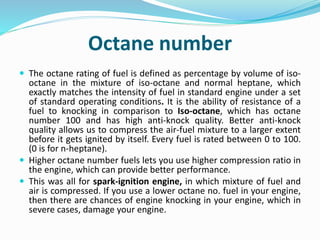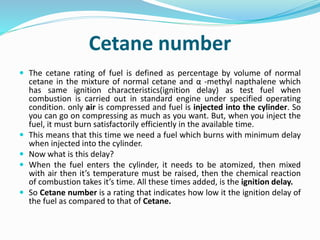1 sur 12

### Calorific Value Lecture 3, Fuel Tech-ll.pptx

• 1. .
• 2. 1. Calorie: It is the amount of heat required to increase the temperature of 1 gram or water through one degree centigrade. 2. Kilocalorie: This is the unit of heat in metric system, and is defined as “the quantity of heat required to raise the temperature of one kilogram of water through one degree centigrade”. 1 k.cal = 1000 cal 3. British thermal unit (B.Th.U.): This is the unit of heat in English system. It is defined as “the quantity of heat required to increase the temperature of one pound of water through one degree Fahrenheit
• 3. 4. Centigrade heat unit (C.H.U.): It is the quantity of heat required to raise the temperature of one pound of water through one degree centigrade. Inter conversion of various units: 1 k.cal = 1000 cal = 3.968 B.T.U. = 2.2 C.H.U. Units of calorific value: The calorific value is generally, expressed in “calorie / gram (cal/g)” or “kilocalorie / kg (kcal/kg)” or “British thermal unit / lb (B.T.U/lb)” in case of solid or liquid fuels. In case of gaseous fuels the units used are “kilocalorie / cubic metre (kcal/m3)” or “B.T.U/ft3
• 4.  HIGHER CALORIFIC VALUE (HCV) Higher calorific value of a fuel portion is defined as the amount of heat evolved when a unit weight (or volume in the case of gaseous fuels) of the fuel is completely burnt and the products of combustion cooled to the normal conditions (with water vapor condensed as a result). The heat contained in the water vapor must be recovered in the condensation process. it is also called Gross Calorific Value (GCV), and Higher Heating Value (HHV)  LOWER CALORIFIC VALUE (LCV) Lower calorific value of a fuel portion is defined as the amount of heat evolved when a unit weight (or volume in the case of gaseous fuels) of the fuel is completely burnt and water vapor leaves with the combustion products without being condensed. It is also called Net Calorific Value (NCV), and Lower Heating Value (LHV).
• 5. Determination of Calorific Value Mass of the fuel = M Kg. Initial temp of the water = t₁ C⁰ Final temp of the water = t₂ C⁰ Change in temp =∆ t = (t₂ – t₁) C⁰ Specific heat of water = S Water equivalent of calorimeter = W Kg. GCV = ( W x S x ∆t )/M J/Kg or GCV = (W + w) x S x ∆t)/M J /Kg NCV = GCV – 0.09 x %H2 x 587 cal/g
• 6. Numerical on Calorific Value Example 1: Calculate calorific value coal samples from the following data. Mass of the coal = 1g. Water equivalent of calorimeter = 2 Kg. Specific heat of water = 4.187 J/Kg/c. Rise of temperature = 4.80C. Example2: A coal sample with 93% carbon, 5% of Hydrogen and 2% Ash is subjected to combustion in a bomb calorimeter. Calculate GCV and NCV Given that; Mass of the coal sample = 0.95g Mass of water in copper calorimeter = 2000g. Water equivalent wt of calorimeter = 700g. Rise in temp = 2.8 0C Latent heat of = 587 cal/g. Specific heat of water = 1 cal/g/0C
• 7. Numerical on Calorific Value Example3: When 0.84g of coal was burnt completely in Bomb calorimeter the increase in temp of 2655 grams of water was 1.850C if the water equivalent calorimeter is 156g Calculate GCV. Example4: Calculate GCV and NCV of a fuel from the following data. Mass of fuel=0.75g, W=350g t =3.020C, Mass of water = 1150, % H2=2.8 Example5: Calculate calorific value of a fuel sample of a coal form the following data. Mass of the coal is 0.6g. Water equivalent wt of calorimeter is 2200g. Specific value 4.187 Kg/KJ/C rise in temperature = 6.520C. Example7: Calculate GCV and NCV of a fuel from the following data. Mass of fuel =0.83g, W=3500g. , W = 385 g, t1 =29.20C, t2 = 26.50C, % H2 = 0.7 and S = 4.2 kj/kg/c
• 8. Knocking In an internal combustion engine, a mixture of gasoline vapour and air is used as a fuel. After the initiation of the combustion reaction, by spark in the cylinder, the flame should spread rapidly and smoothly through the gaseous mixture, thereby the expanding gas drives the piston down the cylinder. The ratio of the gaseous volume in the cylinder at the end of the suction-stoke to the volume at the end of compression-stroke of the piston, is known as the ‘compression ratio’. The efficiency of an internal combustion engine increases with the increase in compression ratio, which is dependent on the nature of the constituents present in the gasoline used. In certain circumstances (due to the presence of some constituents in the gasoline used), the rate of oxidation becomes so great that the last portion of the fuel-air mixture gets ignited instantaneously, producing an explosive violence, known as “knocking”. The knocking results in loss of efficiently.
• 9. Antiknocking Agents Following are the substances added to petrol in order to prevent knocking in I.C. Engines.  Tetra Ethyl led (TEL) Pb(CH2CH3)4, was put into petrol, particles of lead and lead oxide PbO are formed on combustion. This helps the petrol to burn more slowly and smoothly, preventing knocking.  TML Tetra Methyl led.  MTBE Methyl Tertiary Butyl Ether
• 10. Octane number  The octane rating of fuel is defined as percentage by volume of iso- octane in the mixture of iso-octane and normal heptane, which exactly matches the intensity of fuel in standard engine under a set of standard operating conditions. It is the ability of resistance of a fuel to knocking in comparison to Iso-octane, which has octane number 100 and has high anti-knock quality. Better anti-knock quality allows us to compress the air-fuel mixture to a larger extent before it gets ignited by itself. Every fuel is rated between 0 to 100. (0 is for n-heptane).  Higher octane number fuels lets you use higher compression ratio in the engine, which can provide better performance.  This was all for spark-ignition engine, in which mixture of fuel and air is compressed. If you use a lower octane no. fuel in your engine, then there are chances of engine knocking in your engine, which in severe cases, damage your engine.
• 11. Cetane number  The cetane rating of fuel is defined as percentage by volume of normal cetane in the mixture of normal cetane and α -methyl napthalene which has same ignition characteristics(ignition delay) as test fuel when combustion is carried out in standard engine under specified operating condition. only air is compressed and fuel is injected into the cylinder. So you can go on compressing as much as you want. But, when you inject the fuel, it must burn satisfactorily efficiently in the available time.  This means that this time we need a fuel which burns with minimum delay when injected into the cylinder.  Now what is this delay?  When the fuel enters the cylinder, it needs to be atomized, then mixed with air then it’s temperature must be raised, then the chemical reaction of combustion takes it’s time. All these times added, is the ignition delay.  So Cetane number is a rating that indicates how low it the ignition delay of the fuel as compared to that of Cetane.